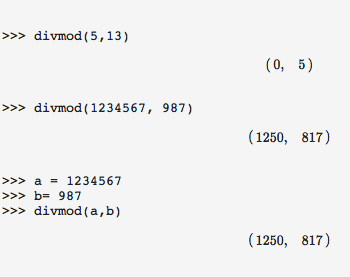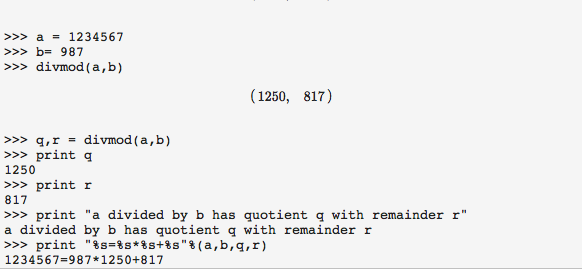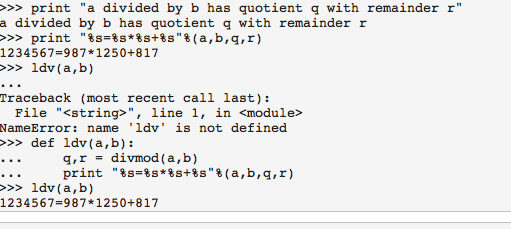Long Division with SymPy
25jun13

\begin{document}
\maketitle
\section{Introduction}
This is a first introduction to using SymPy to learn a smidgeon of Python.
When you open SymPy there is a lot of "chrome" to distract you, and you are
welcome to investigate. But I suggest you do this lesson first. For it
we use only your keyboard, and you should only look at two windows in
display. Under "SymPy Live" the top window is Python's answers to your
input in the bottom window with the >>>. We will refer to the top window
as the \textbf{Journal}, because it records what your and Python have been
doing. The bottom window is the \textbf{Input}, with the >>> prompt.

So, in Journal you can distinguish between what you said and what Python answered.

\section{Lesson}
\subsection{What does divmod do?}When you open SymPy, Python does a bunch of things which you can ignore in this lesson. Don't mess with it.

Python has a primitive function called \texttt{divmod(a,b)} which does long division for you. How it does this is not of any interest here. Afterall, you learned how to do long division a long time ago too. Later, you can try and write a
long division algorithm in Python from scratch, using only subtraction. See the lesson on "anhyphereisis".

First, I made a mistake, thinking the function was called "divmode".
Python says, more politely than some people, that it doesn't understand you.
After writing it correctly, we discovered that the dividend goes first,
in the two arguments of the function, and  divisor goes second. The
twin values of the function is the quotient and the remainder.

\subsection{Variables }We next make Python do a long division you have no desire to do yourself.
I bet you didn't know that $1234567 = 987*1250 + 817$. Now you do.

Then, assign the dividend to a variable named "a". You could have named it
"dividend", but that takes more writing and isn't math, it's computer science.
Note that once the variables have a value you can use them in the function and
get the same result. That's reassuring!

\subsection{Formatted Printing}It would be nice to have Python say what you did, and substitute the values
of the variables into the print out.

The text Python prints out exactly the way you type it in is called a
\textbf{string}, and is always between double quotes. But you do want
to say "q", you want to see 1250. For that you'll need a so-called
formatted string. Here is how to read:\textit{Print the string consisting of a blank number,
followed by an equal sign,
followed by another number, followed by a multiplication star, etc .
To fill in these blanks, evaluate them in the order given.

Exercise 1:
Rewrite the the very first line using what you have just learned to
make it come out "1234567 divided by 987 ...".

\subsection{Extending Python's Function Vocabulary}Finally, we want to extend Python's vocabulary of functions. Let's
call it \texttt{ldv(a,b)} for "long division of $a$ by $b$".

But right away, I made a mistake. I tried to use the
function before I defined it. Poor Python does not understand.

To enter the following into Python you write the first line,
the one with the >>> as prompt, and don't forget the semicolon.
\textbf{WAIT}, don't press the enter/return key. That will give
another error, because of the semicolon. The semicolon tells Python
that there is more to come. You must press \textbf{Shift-Enter}.
This tell Python to give you the three dot prompt, and automatically
indents the next line. Remembering the indentation is a headache
in Python, so SymPy Live does it for you.

You should recognize the next two lines. When you are done writing
a Python program (yup, you have just programmed Python by defining
a new function) press the ordinary enter key. That ends the programming
and you're ready to test your new function.

\end{document}